# Chapter 1. Comparison of Central Tendency of Region A (Asia) and Region B (Europe)Figure 1: Comparison the Central Tendency between two regions

There are three value in the table of Central Tendency including mean, mode and median. According to the table, the mean of total number of deaths (per million population) due to COVID 19 in Asia is 47.329 and much lower than the average value of Europe, which is 190.590. In addition, the median of Asia is 19 while it is 94 in Europe. Finally, the most often figure of death (per million population) in Asia is 0 and much bigger in Europe with 94.

Based on the values of three values above, it is recorded that the Covid-19 in Europe is more serious than in Asia due to the average death is very high (190.590) and the most often number of deaths is 81 while in Asia it is 0.

Chapter 1. Comparison of measures of Variation of Region A (Asia) and Region B (Europe)• The range of Asia (293) is much lower than Europe (846)
• The Asia has Standard Deviation (SD) about 67.409 while it is 209.908 in Europe
• The Coefficient of Variation (CV) of both regions are high in Asia and Europe with 142% and 110% respectively
• The Sample Variance of Asia is about 4543.910 while the Europe has 44061.564

The table of Variation showed the elasticity of two regions Asia and Europe. By considering the isolated data on these two regions, we can assume that Covid-19 situation in Asia is less serious than in Europe which indicates that if people live in an Asian country, the chance of death due to Covid-19 is lower than people living in Europe.

# Chapter 2.              Multiple regression

## 1.         Data set 1: Region A (Asian)

1. Identification of variable type

Dependent variable

• Total number of deaths (per million population) due to COVID 19

Independent variables

• Population of the country (in millions)
• Average rainfall (in mm)
• Medical doctors (per 10,000 people)
• Hospital beds (per 10,000 people)
• Average temperature (in Celsius)

Output:1. Independent variables determination

 Independent variables p-value vs α (=0.05) comparison Determination Average rainfall 0.004 Significant

Regression Equation:

Yˆ = 77.820 – 0.356X1

With

Yˆ:  Total number of deaths (per million population) due to COVID 19

X1:  Average rainfall (in mm)

1. Interpret the regression coefficient

b1 = -0.356 < 0 shows Total number of deaths (per million population) due to COVID 19 decreased by 0.356 for every mm increase in Average rainfall.

1. Interpret the coefficient of determination

r2 = 0.179 = 17.9% ⇒ 17.9% of variation in Total number of deaths (per million population) due to COVID 19 can be explained by variation in in Average rainfall (mm). The remaining 82.1% of variation in Total number of deaths (per million population) due to COVID 19 might because of other factors that we ignore in this study.

## 2.         Data set 1: Region B (Europe)

Identification of variable type

Dependent variable

• Total number of deaths (per million population) due to COVID 19

Independent variables

• Population of the country (in millions)
• Average rainfall (in mm)
• Medical doctors (per 10,000 people)
• Hospital beds (per 10,000 people)
• Average temperature (in Celsius)

Output:b. Independent variables determination

 Independent variables p-value vs α (=0.05) comparison Determination Population of the country (in millions) 0.017 Significant Hospital beds (per 10,000 people) 0.0068 Significant

Regression Equation:

Yˆ = 373.3654 + 2.56X1 -4.57X2

With

Yˆ:  Total number of deaths (per million population) due to COVID 19

X1: Population of the country (in millions)

X2: Hospital beds (per 10,000 people)

1. Interpret the regression coefficient

For every increase in million in the population of the country, the total number of deaths (per million population) due to COVID 19 will increase (B1 >0) with 2.55 people.

For every increase in hospital beds (per 10,000 people), the total number of deaths (per million population) due to COVID 19 will decrease (B2<0) by 4.57 people.

1. Interpret the coefficient of determination

r2 = 0.258 = 25.8% ⇒ 25.8% of variation in Total number of deaths (per million population) due to COVID 19 can be explained by variation of Population of the country (in millions) and Hospital beds (per 10,000 people). The remaining 74.2% of variation in Total number of deaths (per million population) due to COVID 19 might because of other factors that we ignore in this study.

Part4. Team Regression conclusion.

 Significant “independent variables” Value of r2 Asia Average rainfall 0.179 Europe Population of the country (in millions) Hospital beds (per 10,000 people) 0.258

Following the table above regression models provide different significant independent variables. Specifically, the factor that impact  mainly to number of deaths due COVID-19 in Asia belongs to the Average rainfall amount, which in fact related to this tropical region as climate parameters may have a link with the fast transmission rate of COVID-19 (Armitage & Nellums 2020).  Meanwhile in Europe, two independent variables are all significantly effect to Population of the country (in millions) and Hospital beds (per 10,000 people).

According to Rocklöv and Sjödin (2020), high population density catalyses the spread of disease and the main control-strategy of COVID-19 is contact tracing while contact rate is proportional to population density, especially related to the region of highly populated areas such as Europe. Moreover, the reasoning of hospital capacity lack is also take part in increasing the number of deaths during this epidemic when demand of healthcare systems and availability of hospital beds keep expanding in European countries until there exceeded the maximum bed capacity according to Institute for Health Metrics and Evaluation (IHME), results in more infected patents and more death cases within this areas.

# Chapter 3.              Time serires

## 1.         Building trend models for each region

1. A) Region A: Asia

Linear trend (LIN)Independent variables determination

 Independent variables p-value vs α (=0.05) comparison Determination Time period 2.87E-31 Significant

Linear trend formula

Yˆ = 95.38 + 10.39X1

With

Yˆ:  Total number of deaths (per day) due to COVID 19 of Asia

X1: Time period

Coefficient of significant variable analysis

B1= 95.38 => For increase in unit of time period (one day), the total number of deaths (per day) increase by 95.38

Exponential trend (EXP)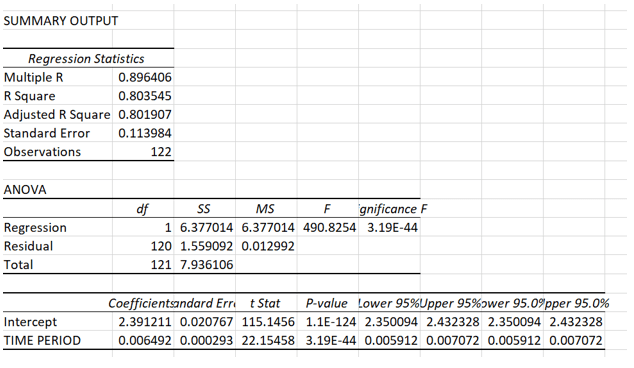Independent variables determination

 Independent variables p-value vs α (=0.05) comparison Determination Time period 3.19E-44 Significant

Exponential trend formula

Yˆ = B0 * B1X1

Log (Yˆ) = B0 + B1* X1

Log (Y^) = 2.391+ 0.0065 * X1

With

Yˆ:  Total number of deaths (per day) due to COVID 19

X1: Time period

Coefficient of significant variable analysis

B1= 2.39 => For increase in unit of time period (one day), the total number of deaths (per day) increase by 2.39

Region B: Europe

Linear trend (LIN)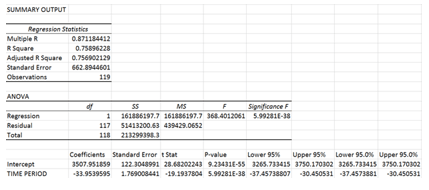Equation:  = 3507.95 – 33.95T

Prediction:

• 29thJuly: T = 120   = 3507.95 – 33.95*120 = -566
• 30thJuly: T = 121   = 3507.95 – 33.95*121 = -600
• 31stJuly: T = 122 = 3507.95 – 33.95*122 = -634

--> Does not make sense.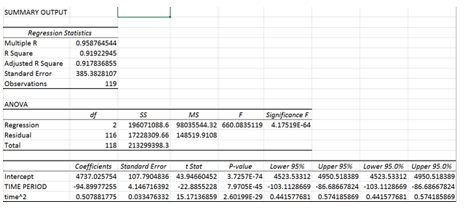Equation:  = 4737.03 – 94.9*T + 0.508*T2

Prediction:

• 29thJuly: T = 120 = 4737.03 – 94.9*120 + 0.508*1202 = 664
• 30thJuly: T = 121 = 4737.03 – 94.9*121 + 0.508*1212 = 692
• 31stJuly: T = 122 = 4737.03 – 94.9*122 + 0.508*1222 = 750

Exponential trend (EXP)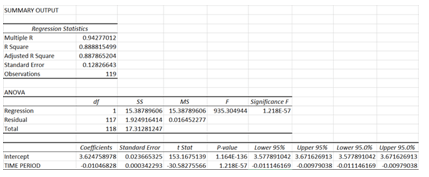Equation:  = 3.62 + (-0.01) *T          (linear form = 103.62-0.01*T                       (non-linear form)

Prediction:

• 29thJuly: T = 120 = 1062-0.01*120 = 264
• 30thJuly: T = 121 = 1062-0.01*121 = 258
• 31stJuly: T = 122 = 1062-0.01*122 = 252

## 2.          Error calculation, MAD and SSE of LIN, QUA and EXP:

With the smallest Error number, MAD and SSE, the deaths per day in Europe, in future, will increase or decrease according to the Exponential trend.

1. Trend model recommendations
2. A) Region A: Asia
 Linear trend model Exponential trend model R square 0.677 0.803

According to the table above, Exponential’s R Square is higher than those of Linear. In other words, 80.3 % of variation in the total number of deaths per day could be explained by the variation of time period (exponential trend model), while this figure of linear trend model is only 67,7%. Therefore, using the exponential trend model will give better and more accurate prediction about the number of deaths due to COVID-19 in Asia.

1. B) Region B: Europe
 Quadratic trend model Exponential trend model R Square 0.919 0.889

After calculating, the output given by linear testing is not make sense. Hence, it is essential to do more mathematic testing, which is R Square and Standard Error.

As can be seen, the output of Error number, MAD and SSE is really small. As a result, in future, the figures will grow up or drop down due to the Exponential trend.

According to the R square comparison, number of Quadratic is a little bit higher than Exponential’s (0.919 and 0.889 respectively). However, looking at the Standard Error, we can conclude that the data of Exponential is more closely to the accurate number. To sum up, Exponential trend is the changing trend of number of deaths due to COVID-19.3

## 3.         Prediction number of deaths due to COVID 19

Application of Exponential model in real life practices might causes some people to against as it remain a very simple one. Nonetheless, we hope that model, which is statistically relevant, can provide informative data. In particular, by the end of September, the exponential model predicts that the European region will be suffered by another swing of the pandemic, predicted mortality cases in three last days of September is 417, 402 and 414 respectively. Whereas, in the case of Asian, the situation is predicted to be worsen than previous months. The number of deaths due to Corona virus in this area is1302 in September 28, 1393 in September 29 and 1281 in September 30.

# Chapter 4.              Time series conclusion

Figure 6‑1 display impacts of Corona virus on human lives in two studied regions. As can be seen in the figure, the overall trend of new deaths per day in two regions follow opposite direction. In European region, though start with very high number of deaths caused by Corona virus per day, yet, the overall situation had been improved, evidenced by consistent decline through May to July. The Asia region, however, established strict policies during pandemic since the lesson of China was very new and considerable. Thereby, the general circumstance in Asia seems to be in better condition than that of Europe. Still, there was a big swing in the middle of July, when the reported numbers of deaths sour up to more than 250 cases per day, signaled a risk of second outbreak in this area.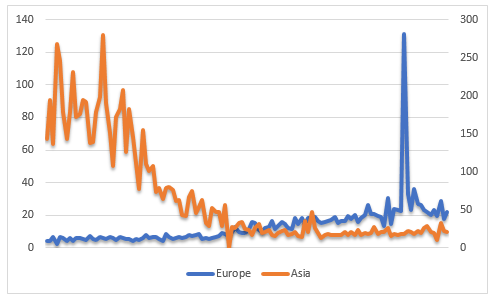Figure 6‑1: Number of deaths per million population from 01/04-31/07/2020

Though the overall trend of two region are clear, nonetheless, there is other factor contributing to movement of two series. Regarding given regions, the cyclical effects seem to be a major components, illustrated by repeated up and down patterns of both regions. Moreover, European and Asia are both display rounded pattern. Due to this motivation, we suggest that the exponential model has potential to be the most appropriate model because it considers for both the overall trend effects and bounded movement. Thus, the exponential model is the best fit model for prediction of Covid impacts on human lives. Further, combined effects of qualitative assessment and quantitative numbers above, we conclude that Quadratic model would be the best model should be implemented in forecasting Corona consequences in global context.

# Chapter 5. Conclusion

In summary, by applying the regression model for both region A (Asia) and region B (Europe), the factors that mainly effected on the total death of Covid-19 are different between two regions. In the Asia, the average rainfall with 17.9% of variation in total number of deaths (per million population) due to COVID 19 can be explained by variation in in Average rainfall (mm) and for every mm increased in average rainfall, the total number of deaths due to Covid-19 would be decreased by 0.356. In contrast, the Population of the country (in millions) and Hospital beds (per 10,000 people) are the two main factor that impact the number of deaths due to COVID 19 in Europe with 25.8% of variation in total number of deaths due to COVID 19 can be explained by these two factors. However, the population and the hospital beds had the opposite impact in the number of deaths: for every million people increased in population, the number of deaths (per million people) will also increase 2.55. In contrast, for every increase in hospital beds (per 10,000 people), the death cases (per million people) would decline 4.57.

As analyzed and stated previously, based on the final model, the number of deaths due to COVID-19 by the end of the year 2020 is predicted to increase for both Europe and Asia with 946.78 and 42.59 new deaths per day consecutively.

Suggestion

The impacts of Sars-Covi 2 on human lives can potentially reflect by Quadratic mode. This model is superior to other proposed models in which it has lowest MAD and SSE, those figures describe preciseness of the model. Adversely, if the quadratic is true, future situation of EU and Asia is predicted to be worse than that at 31 July. By the end of 2020, quadratic model predicts 42.59 new deaths per day in Asia. The circumstance in European region even worsens, under the quadratic prediction, the corona virus will return and create a second outbreak, and the number of deaths is expected to be 946.78 at 31/12/2020.

Our multiple regression model point to the inability of current independent variables in explaining number of deaths caused by Covid 19 pandemic. If we would not be restricted by time and access to data, two other variables that we believe that would have potential powers to explain the dependent variable are population density and current health of infected patient. Regarding the first variable, the role of population density is recognized in previous literatures (Annas et al., 2020; Weinstein et al., 2020). Long incubation of corona virus increases the risk of transmission as many infected patient cannot recognize any substantial symptoms. The risk of cross contamination is even higher in crowded area, where people have chances to contact regularly. Moreover, regarding the second variable, we believe that patient having previous disease such as cancer, respiratory issue and diabetes tend to expose to higher risk of mortality (Assaad et al., 2020). Those patients have lower resistant power to counter adverse effects of Corona virus, thus, have low possibility to recover.

Chia sẻ bài viết